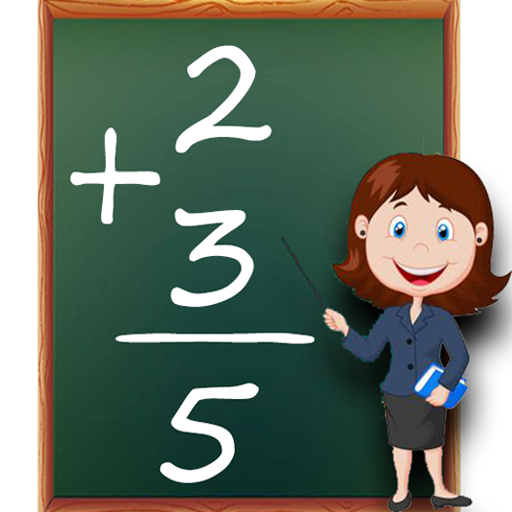### Description

Math Games is a free learning game designed for people who like numbers and math. The best math practice game, this game can exercise your brain, increase your logic and judgment.
A very small math application! Addition, subtraction, multiplication, division, and multiplication tables are all in one application.

Features:
# Subtraction games subtracting numbers to solve the equations.
# Multiplication games multiplication tables learning and duel play mode.
# Division games practice and learn division tables.
# Maths times tables and hard games on maths.

Perform mathematical calculations by simple addition, subtraction, multiplication, and division.

Primary: Games

Secondary: Education

### Total Ratings

This app has not yet been rated.

### Screenshots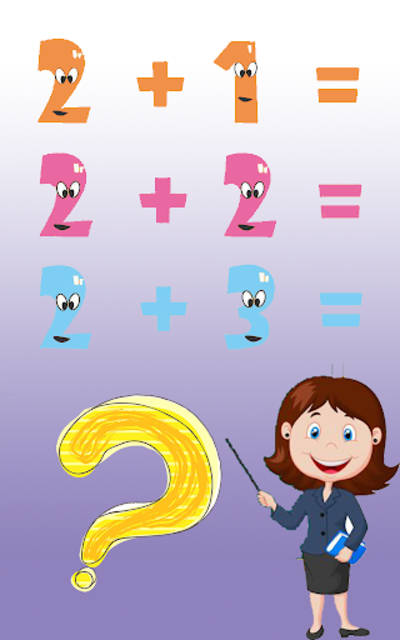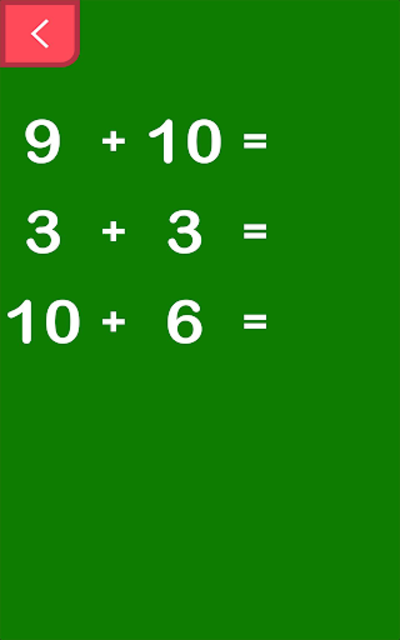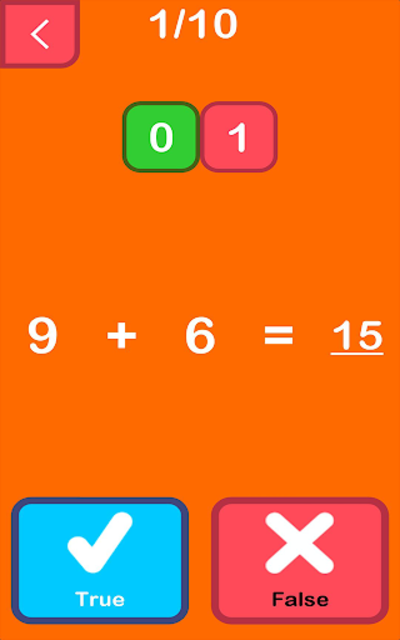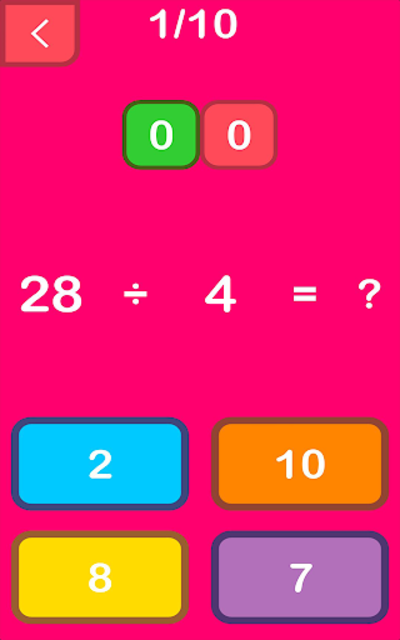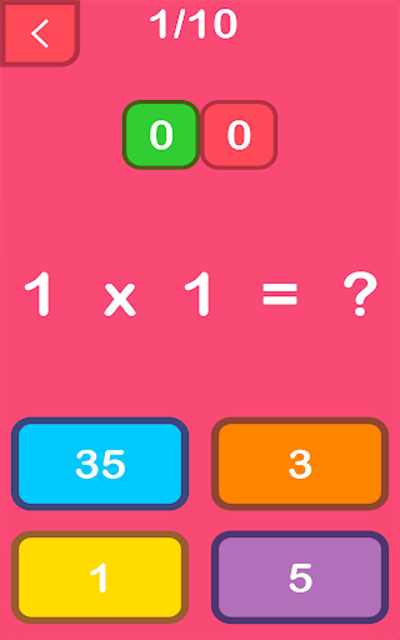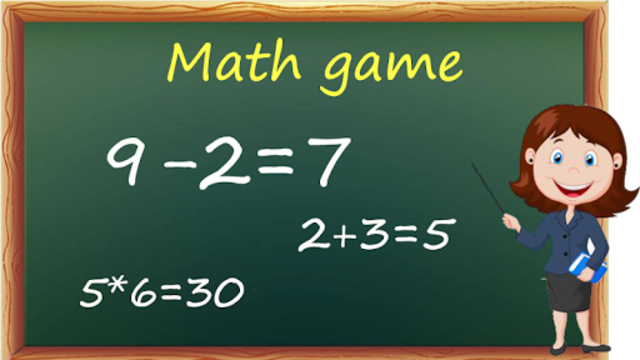### Version History

Launched Jun 27, 2019 (7 months ago).

Releasing new versions every 25 days, on average.

 Dec 152019 (Current)Version 1.0.8-New math learning game in add, subtract, multiply , divide. Nov 242019 Version 1.0.7-New math learning game in add, subtract, multiply , divide. Nov 042019 Version 1.0.6-New math learning game in add, subtract, multiply , divide. Oct 122019 Version 1.0.5-New math learning game in add, subtract, multiply , divide. Sep 262019 Version 1.0.4-New math learning game in add, subtract, multiply , divide. Sep 212019 Version 1.0.2-New math learning game in add, subtract, multiply , divide. Sep 122019 Version 1.0.1-New math learning game in add, subtract, multiply , divide. Jun 262019 Version 1.0.0-New math learning game in add, subtract, multiply , divide. Click to see more